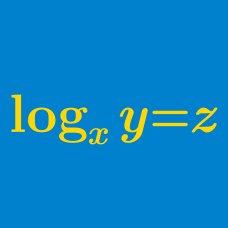Algebra

Logarithmic Functions: Level 3 Challenges

$\large \log_{\sqrt{x}} \left( \sqrt{x\sqrt{x\sqrt{x\sqrt{x}}}} \right) = \, ?$

• Clarification: $x$ is a positive real number and $x \neq 1.$

How many digits does the number $2^{1000}$ contain?

You are given that $\log_{10} 2 = 0.3010$ correct up to 4 decimal places.

$\Large x ^{\log_{10} x } = 100x$

How many real solutions are there to the above equation?

$\Large\begin{cases} \log_x w & = & 24 \\ \log_y w & = & 40 \\ \log_{xyz} w & = & 12 \end{cases}$

If $x,y,z$ are real numbers greater than 1 and $w$ is a positive number satisfying the system above, then find the value of $\left (\log_w z \right)^{-1}$.

Given that

$\log_2(\log_8x)=\log_8(\log_2x),$

find the value of $(\log_2x)^2$.

×